# Samacheer Kalvi 12th Physics Solutions Chapter 7 Dual Nature of Radiation and Matter

Students who wish to prepare the Samacheer Kalvi Class 12th Physics Solutions Subject Chapter 7 Dual Nature of Radiation and Matter can rely on the Tamilnadu State Board Solutions for Class 12th Physics Solutions Chapter 7 Dual Nature of Radiation and Matter Questions and Answers prevailing. Become perfect with the concepts of Samacheer Kalvi Class 12th Physics Solutions Chapter 7 Dual Nature of Radiation and Matter Questions and Answers and score better grades in your exams. Detailed Solutions are provided to the concepts by experts keeping in mind the latest edition textbooks and syllabus.

## Tamilnadu Samacheer Kalvi 12th Physics Solutions Chapter 7 Dual Nature of Radiation and Matter

Ace up your preparation by referring to the Samacheer Kalvi Class 12th Physics Solutions Chapter 7 Dual Nature of Radiation and Matter and learn all the topics within. Click on the topic you want to prepare from the Class 12th Chapter 7 Dual Nature of Radiation and Matter Questions and Answers and prepare it easily. You can understand your strengths and weaknesses by practicing the Questions in Samacheer Kalvi Class 12th Physics Solutions PDF.

### Samacheer Kalvi 12th Physics Dual Nature of Radiation and Matter Textual Evaluation Solved

Samacheer Kalvi 12th Physics Dual Nature of Radiation and Matter Multiple Choice Questions

Question 1.
The wavelength λe of an electron and λp of a photon of same energy E are related by …….. .
(a) λp ∝ λe
(b) λp ∝ $$\sqrt { { \lambda }_{ e } }$$
(c) λp ∝ $$\frac { 1 }{ \sqrt { { \lambda }_{ e } } }$$
(d) λp ∝ $${ \lambda }_{ e }^{ 2 }$$
(d) λp ∝ $${ \lambda }_{ e }^{ 2 }$$
Hint:
de broglie wavelength of electron, λe = $$\frac { h }{ \sqrt { 2mE } }$$
∴ ie λe ∝ $$\frac { 1 }{ \sqrt { E } }$$ ⇒ $${ \lambda }_{ e }^{ 2 }$$ ∝ $$\frac { 1 }{ E }$$ …… (1)
de-Broglie wavelength of proton
λp = $$\frac { hc }{ E }$$
λp ∝ $$\frac { 1 }{ E }$$ …… (2)
From (1) and (2)
$${ \lambda }_{ e }^{ 2 }$$ ∝ λp i.e., λp ∝ $${ \lambda }_{ e }^{ 2 }$$

Question 2.
In an electron microscope, the electrons are accelerated by a voltage of 14 kV. If the voltage is changed to 224 kV, then the de Broglie wavelength associated with the electrons would …….. .
(a) increase by 2 times
(b) decrease by 2 times
(c) decrease by 4 times
(d) increase by 4 times
(c) decrease by 4 times
Hint:
At Voltage, V = 14 kV
de-Broglie wavelength of electron,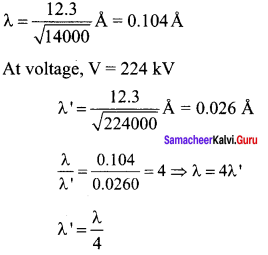the de-Broglie wavelength of an electron is decreased by 4 times

Question 3.
A particle of mass 3 x 10-6 g has the same wavelength as an electron moving with a velocity 6 x 106 ms-1 The velocity of the particle is ………
(a) 1.82 x 10-18 ms-1
(b) 9 x 10-2 ms-1
(c) 3 x 10-31 ms-1
(d) 1.82 x 10-15 ms-1
(d) 1.82 x 10-15 ms-1
Hint: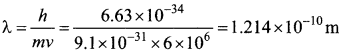Velocity of the particle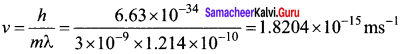Question 4.
When a metallic surface is illuminated with radiation of wavelength λ, the stopping potential is V. If the same surface is illuminated with radiation of wavelength 2λ, the stopping potential is $$\frac { V }{ 4 }$$. The threshold wavelength for the metallic surface is ………..
(a) 4λ
(b) 5λ
(c) $$\frac { 5 }{ 2 }$$ λ
(d) 3λ
(d) 3λ
Hint: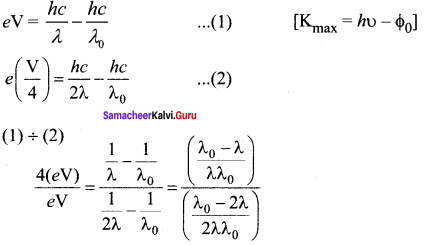On solving we get, λ0 = 3λ

Question 5.
If light of wavelength 330 nm is incident on metal with work function 3.55 eV, the electrons are emitted. Then the wavelength of the emitted electron is (Take h = 6.6 x 10-34 Js) ……….
(a) < 2.75 x 10-9 m
(b) ≥ 2.75 x 10-9 m
(c) < 2.75 x 10-12um
(d) ≤ 2.75 x 10-10um
(a) < 2.75 x 10-9 m
Hint:
Maximum KE of emitted electron is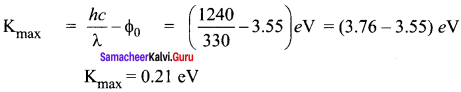de-Broglie wavelength of emitted electron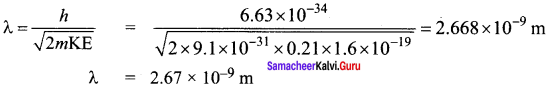The Two wavelength of the emitted electron is < 2.75 x 10-9 m

Question 6.
A photoelectric surface is illuminated successively by monochromatic light of wavelength λ and $$\frac { λ }{ 2 }$$ . If the maximum kinetic energy of the emitted photoelectrons in the second case is 3 times that in the first case, the work function at the surface of material is …….. .
(a) $$\frac { hc }{ λ }$$
(b) $$\frac { 2hc }{ λ }$$
(c) $$\frac { hc }{ 3λ }$$
(d) $$\frac { hc }{ 2λ }$$
(d) $$\frac { hc }{ 2λ }$$
Hint:
KE1 = $$\frac { hc }{ λ }$$ – Φ ……. (2)
3KE1 = $$\frac { 2hc }{ λ }$$ – Φ
KE1 = $$\frac { 2hc }{ 3λ }$$ – $$\frac { Φ }{ 3λ}$$ ….. (2)
Equating (1) and (2)
$$\frac { hc }{ λ }$$ – Φ = $$\frac { 2hc }{ 3λ }$$ – $$\frac { Φ }{ 3λ}$$
$$\frac { hc }{ 3λ }$$ = $$\frac { 2Φ }{ 3λ}$$ ⇒ Φ = $$\frac { hc }{ 2λ }$$

Question 7.
In photoelectric emission, a radiation whose frequency is 4 times threshold frequency of a certain metal is incident on the metal. Th en the maximum possible velocity of the emitted electron will be ……….. .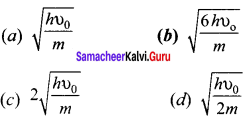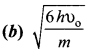Hint:
From Einstein’s photoelectric equation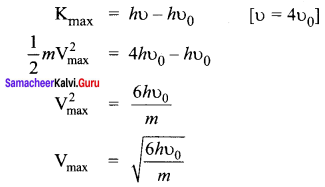Question 8.
Two radiations with photon energies 0.9 eV and 3.3 eV respectively are falling on a metallic surface successively. If the work function of the metal is 0.6 eV, then the ratio of maximum speeds of emitted electrons will be ………..
(a) 1 : 4
(b) 1 : 3
(c) 1 : 1
(d) 1 : 9
(b) 1 : 3
Hint: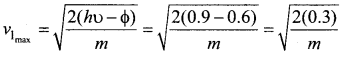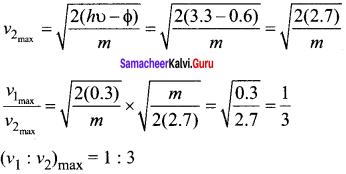Question 9.
A light source of wavelength 520 nm emits 1.04 x 1015 photons per second while the second source of 460 nm produces 1.38 x 1015 photons per second. Then the ratio of power of second source to that of first source is ……… .
(a) 1.00
(b) 1.02
(c) 1.5
(d) 0.98
(c) 1.5
Hint:
Power: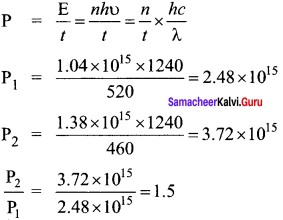Question 10.
The mean wavelength of light from sun is taken to be 550 nm and its mean power is 3.8 x 1026 W. The number of photons received by the human eye per second on the average from sunlight is of the order of ………. .
(a) 1045
(b) 1042
(c) 1054
(d) 1051
(a) 1045
Hint: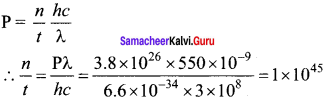Question 11.
The threshold wavelength for a metal surface whose photoelectric work function is 3.313 eV is
(a) 4125 Å
(b) 3750 Å
(c) 6000 Å
(d) 2062.5 Å
(b) 3750 Å
Hint: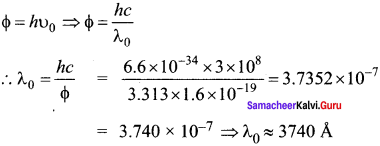Question 12.
A light of wavelength 500 nm is incident on a sensitive plate of photoelectric work function 1.235 eV. The kinetic energy of the photo electrons emitted is be (Take h = 6.6 x 10-34 Js)
(a) 0.58 eV
(b) 2.48 eV
(c) 1.24 eV
(d) 1.16 eV
(c) 1.24 eV
Hint: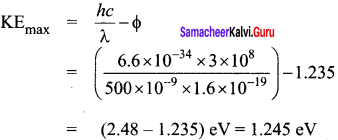Question 13.
Photons of wavelength λ are incident on a metal. The most energetic electrons ejected from the metal are bent into a circular arc of radius R by a perpendicular magnetic field having magnitude B. The work function of the metal is ……… .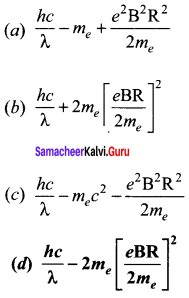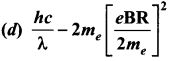Hint:
Magnetic lorentz force = Centripetal force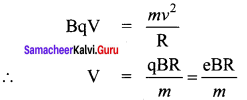From Einstein’s photo electric equation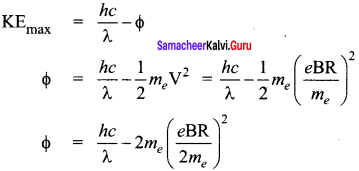Question 14.
The work functions for metals A, B and C are 1.92 eV, 2.0 eV and 5.0 eV respectively. The metals which will emit photoelectrons for a radiation of wavelength 4100 Å is/are ………. .
(a) A only
(b) both A and B
(c) all these metals
(d) none
(b) both A and B
Hint: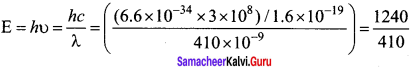E = 3.04 eV
Since energy of incident radiation is greater than the work function of metals A and B. So metal A and B will emit photoelectrons.

Question 15.
Emission of electrons by the absorption of heat energy is called ……… emission.
(a) photoelectric
(b) field
(c) thermionic
(d) secondary
(c) thermionic

Samacheer Kalvi 12th Physics Dual Nature of Radiation and Matter Short Answer Questions

Question 1.
Why do metals have a large number of free electrons?

• In metals, the electrons in the outermost shells are loosely bound to the nucleus.
• At room temperature, there are a large number of free electrons moving inside the metal in a random manner.

Question 2.
Define work function of a metal. Give its unit.
The minimum energy needed for an electron to escape from the metal surface is called work function of that metal. It’s unit is electron volt (eV).

Question 3.
What is photoelectric effect?
The ejection of electrons from a metal plate when illuminated by light or any other electromagnetic radiation of suitable wavelength (or frequency) is called photoelectric effect.

Question 4.
How does photocurrent vary with the intensity of the incident light?
The number of electrons emitted per second is directly proportional to the intensity of the incident light.

Question 5.
Give the definition of intensity of light and its unit.
Intensity of light refer to the strength or brightness or amount of light produced by a specific source. It’s unit is candela (cd)

Question 6.
How will you define threshold frequency?
For a given surface, the emission of photoelectrons takes place only if the frequency of incident light is greater than a certain minimum frequency called the threshold frequency.

Question 7.
What is a photocell? Mention the different types of photocells.
The photocell is a device that converts light energy into electrical energy.
Three types of photocells are

1. Photo emissive cell
2. Photovoltaic cell
3. Photoconductive cell

Question 8.
Write the expression for the de Broglie wavelength associated with a charged particle of charge q and mass m, when it is accelerated through a potential V.
An electron of mass m is accelerated through a potential difference of V volt. The kinetic energy acquired by the electron is given by
$$\frac { 1 }{ 2 }$$ mv2 = eV
Therefore, the speed v of the electron is v = $$\sqrt { \frac { 2ev }{ m } }$$
Hence, the de Broglie wavelength of the electron is λ = $$\frac { h }{ mv }$$ = $$\frac { h }{ \sqrt { 2emV } }$$

Question 9.
State de Broglie hypothesis.
All matter particles like electrons, protons, neutrons in motion are associated with waves. These waves are called de Broglie waves (or) matter waves.

Question 10.
Why we do not see the wave properties of a baseball?
Due to the large mass of a baseball, the de Broglie wavelength
[λ = $$\frac { h }{ mv }$$] associated with a moving baseball is very small. Hence its wave nature is not visible.

Question 11.
A proton and an electron have the same kinetic energy. Which one has a greater de Broglie wavelength? Justify.
1. We know that
λ = $$\frac{\mathrm{h}}{\sqrt{2 \mathrm{mK}}}$$;
K = $$\frac{1}{2}$$ mv2 then v = $$\sqrt{\frac{2 \mathrm{~K}}{\mathrm{~m}}}$$

2. Since mp >>> me, having lower mass the electron has the larger De Broglie wavelength.

Question 12.
Write the relationship of de Broglie wavelength λ associated with a particle of mass m in terms of its kinetic energy K.
Kinetic energy of the particle, K = $$\frac { 1 }{ 2 }$$ mv2 = $$\frac { { P }^{ 2 } }{ 2m }$$
p = $$\sqrt { 2mK }$$
de-Broglie wavelength of the particle λ = $$\frac { h }{ p }$$ = $$\frac { h }{ \sqrt { 2mK } }$$

Question 13.
Name an experiment which shows wave nature of the electron. Which phenomenon was observed in this experiment using an electron beam?

• Electron microscope shows wave nature of the electron.
• Electron beam having lower wavelength gives higher resolving power.

Question 14.
An electron and an alpha particle have same kinetic energy. How are the de Broglie wavelengths associated with them related?
[λ = $$\frac { h }{ p }$$]
Kinetic energy of the particle K = $$\frac { 1 }{ 2 }$$ mv2 = $$\frac { { P }^{ 2 } }{ 2m }$$ = $$\frac { { h }^{ 2 } }{ 2m{ \lambda }^{ 2 } }$$
i.e. λ = $$\frac { h }{ \sqrt { 2mK } }$$ ; λ ∝ $$\frac { 1 }{ \sqrt { m} }$$
$$\frac { { \lambda }_{ e } }{ { \lambda }_{ \alpha } }$$ = $$\sqrt { \frac { { m }_{ \alpha } }{ { m }_{ e } } }$$

Samacheer Kalvi 12th Physics Dual Nature of Radiation and Matter Long Answer Questions

Question 1.
What do you mean by electron emission? Explain briefly various methods of electron emission.
Electron emission:
1. Free electrons possess some kinetic energy and this energy is different for different electrons. The kinetic energy of the free electrons is not sufficient to overcome the surface barrier.

2. Whenever additional energy is given to the free electrons, they will have sufficient energy to cross the surface barrier. And they escape from the metallic surface.

3. The liberation of electrons from any surface of a substance is called electron emission.

There are mainly four types of electron emission which are given below.
(i) Thermionic emission:
When a metal is heated to a high temperature, the free electrons on the surface of the metal get sufficient energy in the form of thermal energy so that they are emitted from the metallic surface. This type of emission is known as thermionic emission.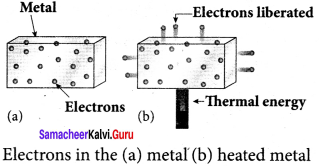The intensity of the thermionic emission (the number of electrons emitted) depends on the metal used and its temperature.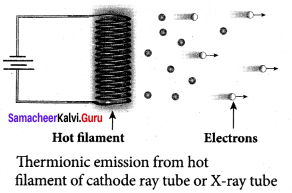Examples: cathode ray tubes, electron microscopes, X-ray tubes etc.

(ii) Field emission:
Electric field emission occurs when a very strong electric field is applied across the metal. This strong field pulls the free electrons and helps them to overcome the surface barrier of the metal.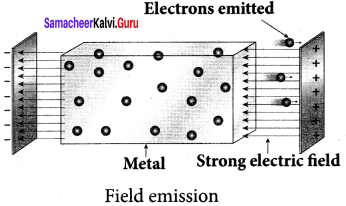Examples: Field emission scanning electron microscopes, field-emission display etc.

(iii) Photoelectric emission:
When electromagnetic radiation of suitable frequency is incident on the surface of the metal, the energy is transferred from the radiation to the free electrons. Hence, the free electrons get sufficient energy to cross the surface barrier and the photoelectric emission takes place. The number of electrons emitted depends on the intensity of the incident radiation.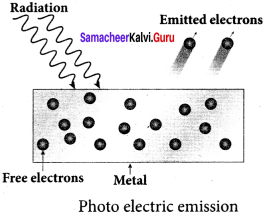Examples: Photodiodes, photoelectric cells etc.

(iv) Secondary emission:
When a beam of fast-moving electrons strikes the surface of the metal, the kinetic energy of the striking electrons is transferred to the free electrons on the metal surface. Thus the free electrons get sufficient kinetic energy so that the secondary emission of an electron occurs.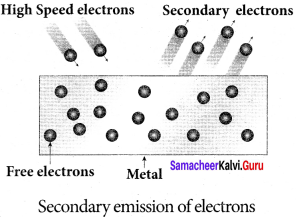Examples: Image intensifies, photomultiplier tubes, etc.

Question 2.
Briefly discuss the observations of Hertz, Hallwachs, and Lenard.
Hertz observation:
1. In 1887, Heinrich Hertz first became successful in generating and detecting electromagnetic waves with his high voltage induction coil to cause a spark discharge between two metallic spheres.

2. When a spark is formed, the charges will oscillate back and forth rapidly and the electromagnetic waves are produced.

3. The electromagnetic waves thus produced were detected by a detector that has a copper wire bent in the shape of a circle. Although the detection of waves is successful, there is a problem in observing the tiny spark produced in the detector.

4. In order to improve the visibility of the spark, Hertz made many attempts and finally noticed an important thing that the small detector spark became more vigorous when it was exposed to ultraviolet light.

5. The reason for this behaviour of the spark was not known at that time. Later it was found that it is due to the photoelectric emission.

6. Whenever ultraviolet light is incident on the metallic sphere, the electrons on the outer surface are emitted which caused the spark to be more vigorous.

Hallwachs’ observation: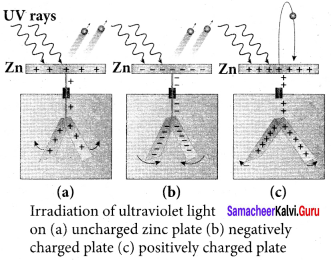8. In 1888, Wilhelm Hallwachs, a German physicist, confirmed that the strange behaviour of the spark is due to the action of ultraviolet light with his simple experiment.

9. A clean circular plate of zinc is mounted on an insulating stand and is attached to a gold leaf electroscope by a wire. When the uncharged zinc plate is irradiated by ultraviolet light from an arc lamp, it becomes positively charged and the leaves will open.

10. Further, if the negatively charged zinc plate is exposed to ultraviolet light, the leaves will close as the charges leaked away quickly. If the plate is positively charged, it becomes more positive upon UV rays irradiation and the leaves will open further.

11. From these observations, it was concluded that negatively charged electrons were emitted from the zinc plate under the action of ultraviolet light.

Lenard’s observation:
1. In 1902, Lenard studied this electron emission phenomenon in detail. The apparatus consists of two metallic plates A and C placed in an evacuated quartz bulb. The galvanometer G and battery B are connected in the circuit.

2. When ultraviolet light is incident on the negative plate C, an electric current flows in the circuit that is indicated by the deflection in the galvanometer. On other hand, if the positive plate is irradiated by the ultraviolet light, no current is observed in the circuit.

3. From these observations, it is concluded that when ultraviolet light falls on the negative plate, electrons are ejected from it which are attracted by positive plate A. On reaching the positive plate through the evacuated bulb, the circuit is completed and the current flows in it.

4. Thus, the ultraviolet light falling on the negative plate causes the electron emission from the surface of the plate.

Question 3.
Explain the effect of potential difference on photoelectric current.
Effect of potential difference on the photoelectric current:
1.  To study the effect of potential difference V between the electrodes on photoelectric current, the frequency and intensity of the incident light are kept constant. Initially, the potential of A is kept positive with respect to C and the cathode is irradiated with the given light.

2. Now, the potential of A is increased and the corresponding photocurrent is noted. As the potential of A is increased, photocurrent is also increased. However, a stage is reached where photocurrent reaches a saturation value (saturation current) at which all the photoelectrons from C are collected by A. This is represented by the flat portion of the graph between the potential of A and photocurrent.

3. When a negative (retarding) potential is applied to A with respect to C, the current does not immediately drop to zero because the photoelectrons are emitted with some definite and different kinetic energies.

4. The kinetic energy of some of the photoelectrons is such that they could overcome the retarding electric field and reach electrode A.

5. When the negative (retarding) potential of A is gradually increased, the photocurrent starts to decrease because more and more photoelectrons are being repelled away from reaching the electrode A. The photocurrent becomes zero at a particular negative potential V0, called stopping or cut-off potential.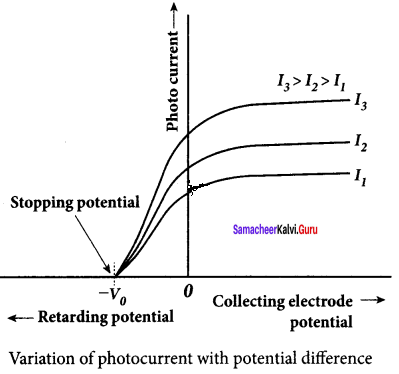6. Stopping potential is that the value of the negative (retarding) potential given to the collecting electrode A which is just sufficient to stop the most energetic photoelectrons emitted and make the photocurrent zero.

7. At the stopping potential, even the most energetic electron is brought to rest. Therefore, the initial kinetic energy of the fastest electron (Kmax ) is equal to the work done by the stopping potential to stop it (eV0 ).
Kmax = $$\frac { 1 }{ 2 }$$ $${ mv }_{ max }^{ 2 }$$ = eV0 …. (1)
Where vmax is the maximum speed of the emitted photoelectron.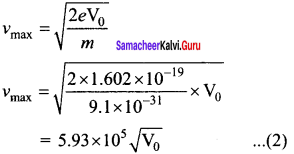= 5.93 x 105 $$\sqrt { { V }_{ 0 } }$$ …. (2)
From equation (1),
Kmax = eVo (in joule) (or) Kmax = (V0 ) (in eV)

8. From the graph, when the intensity of the incident light alone is increased, the saturation current also increases but the value of V0 remains constant.

9. Thus, for a given frequency of the incident light, the stopping potential is independent of the intensity of the incident light. This also implies that the maximum kinetic energy of the photoelectrons is independent of the intensity of the incident light.

Question 4.
Explain how the frequency of incident light varies with stopping potential.
Effect of frequency of incident light on stopping potential:
1. To study the effect of the frequency of incident light on stopping potential, the intensity of the incident light is kept constant. The variation of photocurrent with the collector electrode potential is studied for radiations of different frequencies and a graph drawn between them. From the graph, it is clear that stopping potential vary over different frequencies of incident light.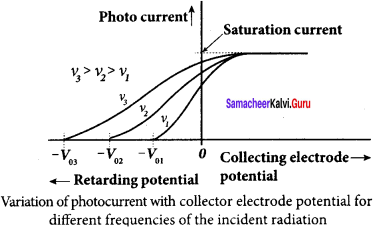2. Greater the frequency of the incident radiation, the larger is the corresponding stopping potential. This implies that as the frequency is increased, the photoelectrons are emitted with greater kinetic energies so that the retarding potential needed to stop the photoelectrons is also greater.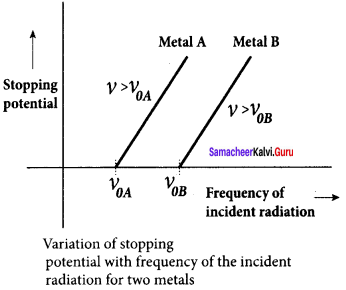3. Now a graph is drawn between frequency and the stopping potential for different metals. From this graph, it is found that stopping potential varies linearly with frequency. Below a certain frequency called threshold frequency, no electrons are emitted; hence stopping potential is zero for that reason. But as the frequency is increased above a threshold value, the stopping potential varies linearly with the frequency of incident light.

Question 5.
List out the laws of the photoelectric effect.
Laws of photoelectric effect:
1. For a given frequency of incident light, the number of photoelectrons emitted is directly proportional to the intensity of the incident light. The saturation current is also directly proportional to the intensity of incident light.

2. Maximum kinetic energy of the photoelectrons is independent of the intensity of the incident light.

3. Maximum kinetic energy of the photoelectrons from a given metal is directly proportional to the frequency of incident light.

4. For a given surface, the emission of photoelectrons takes place only if the frequency of incident light is greater than a certain minimum frequency called the threshold frequency.

5. There is no time lag between the incidence of light and the ejection of photoelectrons.

Question 6.
Explain why the photoelectric effect cannot be explained on the basis of the wave nature of light.

1. According to maxwell’s theory, light is an electromagnetic wave consisting of coupled electric and magnetic oscillations that move with the speed of light which exhibits typical wave behaviour.
2. By the wave theory, the light of greater intensity should impart greater kinetic energy to the liberated electrons. Whereas the maximum kinetic energy of the photoelectrons does not depend on the intensity of the incident light.
3. According to wave theory, if a sufficiently intense beam of light is incident on the surface, electrons will be liberated however low the frequency of the radiation is. But the wave theory fails to explain the existence of threshold frequency.
4. Each electron needs a considerable amount of time to get sufficient energy to overcome the work function and to get liberated from the surface. But photoelectric emission is an almost instantaneous process which could not be explained by the wave theory.
5. Thus, the experimental observations of photoelectric emission could not be explained on the basis of the wave theory of light.

Question 7.
Explain the quantum concept of light.
Concept of quantization of energy:
Max Planck proposed quantum concept in 1900 in order to explain the thermal radiations emitted by a black body and the shape of its radiation curves. According to Planck, matter is composed of a large number of oscillating particles (atoms) which vibrate with different frequencies. Each atomic oscillator – which vibrates with its characteristic frequency – emits or absorbs electromagnetic radiation of the same frequency. It also says that

1. If an oscillator vibrates with frequency v, its energy can have only certain discrete values, given by the equation.
En = nhυ n = 1, 2, 3 ………..
where A is a constant, called Planck’s constant.

2. The oscillators emit or absorb energy in small packets or quanta and the energy of each quantum is E = hυ.
This implies that the energy of the oscillator is quantized – that is, energy is not continuous as believed in the wave picture. This is called the quantization of energy.

Question 8.
Obtain Einstein’s photoelectric equation with the necessary explanation. Einstein’s explanation of photoelectric equation:
1.  When a photon of energy hv is incident on a metal surface, it is completely absorbed by a single electron and the electron is ejected.

2.  In this process, a part of the photon energy is used for the ejection of the electrons from the metal surface (photoelectric work function Φ0) and the remaining energy as the kinetic energy of the ejected electron. From the law of conservation of energy,
hυ = Φ0 + $$\frac { 1 }{ 2 }$$ mv2 …… (1)
where m is the mass of the electron and u its velocity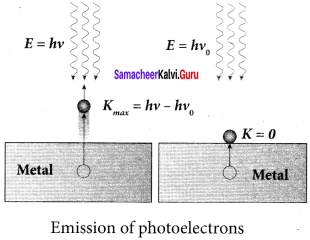3. If we reduce the frequency of the incident light, the speed or kinetic energy of photoelectrons is also reduced. At some frequency V0 of incident radiation, the photoelectrons are ejected with almost zero kinetic energy. Then the equation (1) becomes
0 = Φ0
where vQ is the threshold frequency. By rewriting the equation (1), we get
hυ = hυ0 + $$\frac { 1 }{ 2 }$$ mv2 …… (2)
The equation (2) is known as Einstein’s Photoelectric equation.
If the electron does not lose energy by internal collisions, then it is emitted with maximum kinetic energy Kmax. Then
Kmax = $$\frac { 1 }{ 2 }$$ $${ mv }_{ max }^{ 2 }$$
where nmax is the maximum velocity of the electron ejected. The equation (1) is rearranged as follows:
Kmax = hυ – Φ0

Question 9.
Explain experimentally observed facts of the photoelectric effect with the help of Einstein’s explanation.
The explanation for the photoelectric effect:
The experimentally observed facts of photoelectric effect can be explained with the help of . Einstein’s photoelectric equation.

1. As each incident photon liberates one electron, then the increase of intensity of the light (the number of photons per unit area per unit time) increases the number of electrons emitted thereby increasing the photocurrent. The same has been experimentally observed.

2. From Kmax = hυ – Φ0, it is evident that Kmax is proportional to the frequency of the light and is independent of intensity of the light.

3.  As given in Einstein’s photoelectric equation, there must be minimum energy (equal to the work function of the metal) for incident photons to liberate electrons from the metal surface. Below which, emission of electrons is not possible. Correspondingly, there exists a minimum frequency called threshold frequency below which there is no photoelectric emission.

4. According to the quantum concept, the transfer of photon energy to the electrons is instantaneous so that there is no time lag between the incidence of photons and the ejection of electrons.

Question 10.
Give the construction and working of a photoemissive cell.
Photo emissive cell:
Its working depends on the electron emission from a metal cathode due to irradiation of light or other radiations.
Construction:
1. It consists of an evacuated glass or quartz bulb in which two metallic electrodes – that is, a cathode and an anode are fixed.

2. The cathode C is semi-cylindrical in shape and is coated with a photo-sensitive material. Anode A is a thin rod or wire kept along the axis of the semi-cylindrical cathode.

3. A potential difference is applied between the anode and the cathode through a galvanometer G.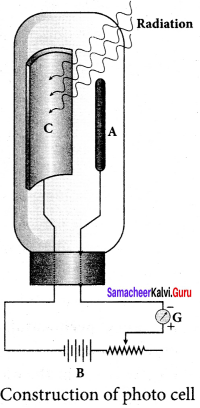Working:
4.  When the cathode is illuminated, electrons are emitted from it. These electrons are attracted by anode and hence a current is produced which is measured by the galvanometer.

5. For a given cathode, the magnitude of the current depends on
(i) the intensity to incident radiation and (ii) the potential difference between anode and cathode.

Question 11.
Derive an expression for de Broglie wavelength of electrons.
An electron of mass m is accelerated through a potential difference of V volt. The kinetic
energy acquired by the electron is given by
$$\frac { 1 }{ 2 }$$ mv2 = evacuated
Therefore, the speed v of the electron is v = $$\sqrt { \frac { 2eV }{ m } }$$
Hence, the de Broglie wavelength of the electron is λ = $$\frac { h }{ mv }$$ = $$\frac { h }{ \sqrt { 2emV } }$$
Substituting the known values in the above equation, we get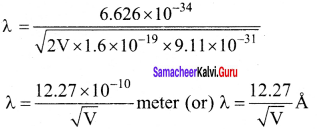For example, if an electron is accelerated through a potential difference of 100V, then its de Broglie wavelength is 1.227 A. Since the kinetic energy of the electron, K = eV, then the de Broglie wavelength associated with electron can be also written as
λ = $$\frac { h }{ \sqrt { 2mK } }$$

Question 12.
Briefly explain the principle and working of an electron microscope.
Electron Microscope:
Principle:
1. This is the direct application of the wave nature of particles. The wave nature of the electron is used in the construction of a microscope called an electron microscope.

2. The resolving power of a microscope is inversely proportional to the wavelength of the radiation used for illuminating the object under study. Higher magnification, as well as higher resolving power, can be obtained by employing the waves of shorter wavelengths.

3. De Broglie wavelength of an electron is very much less than (a few thousand less) that of the visible light being used in optical microscopes.

4. As a result, the microscopes employing de Broglie waves of electrons have very much higher resolving power than an optical microscope.

5. Electron microscopes giving magnification more than 2,00.000 times are common in research laboratories.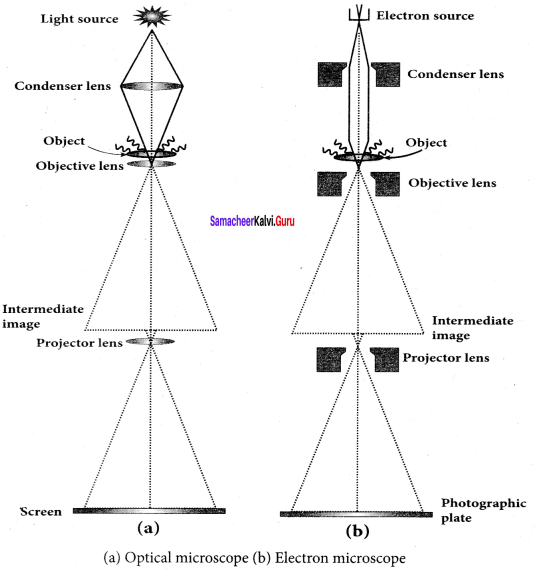Working:
1. The electron beam passing across a suitably arranged either electric or magnetic fields undergoes divergence or convergence thereby focussing of the beam is done.

2. The electrons emitted from the source are accelerated by high potentials. The beam is made parallel by a magnetic condenser lens. When the beam passes through the sample whose magnified image is needed, the beam carries the image of the sample.

3. With the help of a magnetic objective lens and magnetic projector lens system, the magnified image is obtained on the screen. These electron microscopes are being used in almost all branches of science.

Question 13.
Describe briefly Davisson – Germer experiment which demonstrated the wave nature of electrons.
Davisson – Germer experiment:
1. De Broglie hypothesis of matter waves was experimentally confirmed by Clinton Davisson and Lester Germer in 1927. They demonstrated that electron beams are diffracted when they fall on crystalline solids.

2. Since crystal can act as a three-dimensional diffraction grating for matter waves, the electron waves incident on crystals are diffracted off in certain specific directions.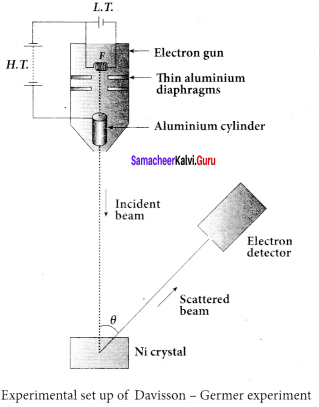3. The filament F is heated by a low tension (L.T.) battery. Electrons are emitted from the hot filament by thermionic emission. They are then accelerated due to the potential difference between the filament and the anode aluminium cylinder by a high tension (H.T.) battery.

4. Electron beam is collimated by using two thin aluminium diaphragms and is allowed to strike a single crystal of Nickel.

5. The electrons scattered by Ni atoms in different directions are received by the electron detector which measures the intensity of scattered electron beam.

6. The detector is rotatable in the plane of the paper so that the angle Φ between the incident
beam and the scattered beam can be changed at our will.

7. The intensity of the scattered electron beam is measured as a function of the angle θ.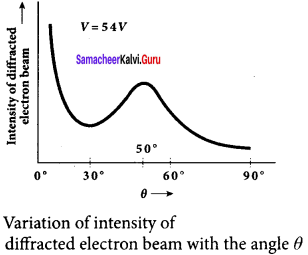8. From the graph shows the variation of intensity of the scattered electrons with the angle 0 for the accelerating voltage of 54V. For a given accelerating voltage V, the scattered wave shows a peak or maximum at an angle of 50° to the incident electron beam.

This peak in intensity is attributed to the constructive interference of electrons diffracted from various atomic layers of the target material.

9. From the known value of interplanar spacing of Nickel, the wavelength of the electron wave has been experimentally calculated as 1.65 Å.

10. The wavelength can also be calculated from de Broglie relation for V = 54 V from equation.
λ = $$\frac { 12.27 }{ \sqrt { V } }$$ Å = $$\frac { 12.27 }{ \sqrt { 54 } }$$
λ = 1.67 Å

11. This value agrees well with the experimentally observed wavelength of 1.65 Å. Thus this experiment directly verifies de Broglie’s hypothesis of the wave nature of moving particles.

Samacheer Kalvi 12th Physics Dual Nature of Radiation and Matter Numerical problems

Question 1.
How many photons per second emanate from a 50 mW laser of 640 nm?
P = 50 mW
λ = 640 nm
h = 6.6 x 10-34 Js
c = 3 x 108 ms-1
Number of photons emanate per second np = $$\frac { P }{ E }$$ =$$\frac { Pλ }{ hc }$$
= $$\frac { 50\times { 10 }^{ 3 }\times 640\times { 10 }^{ -9 } }{ 6.6\times { 10 }^{ -34 }3\times { 10 }^{ 8 } }$$ = $$\frac { 32000\times { 10 }^{ -6 } }{ 19.8\times { 10 }^{ -26 } }$$ = 1616.16 x 10-6
np = 1.61 x 101017 s-1

Question 2.
Calculate the maximum kinetic energy and maximum velocity of the photoelectrons emitted when the stopping potential is 81 V for the photoelectric emission experiment.
V0 = 81 V
e= 1.6 x 10-19 C
m = 9.1 x 10-31 kg
Maximum kinetic energy of electron,
Kmax = eVo
= 1.6 x 10-19 x 81
= 129.6 x 10-19
= 1.29 x 10-17
Kmax = 1.3 x 10-17 J
aximum velocity of photoelectron,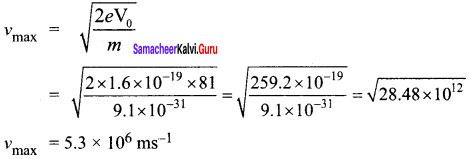Question 3.
Calculate the energies of the photons associated with the following radiation:
(i) violet light of 413 nm
(ii) X-rays of 0.1 nm
(iii) radio waves of 10 m.
h = 6.6 x 10-34 Js
c = 3 x 108 ms-1
Energy of photon, E = hυ
E = $$\frac { hc }{ λ }$$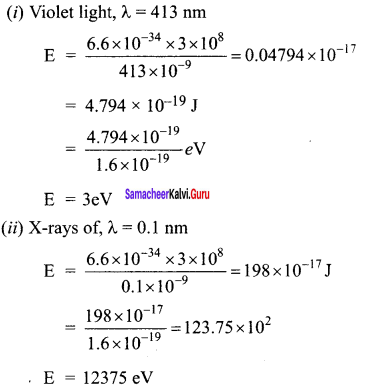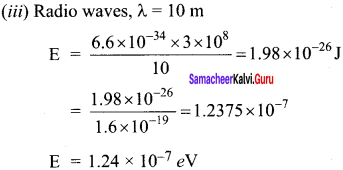Question 4.
A 150 W lamp emits light of mean wavelength of 5500 Å . If the efficiency is 12%, find out the number of photons emitted by the lamp in one second.
P= 150W
λ = 5500 Å
h = 6.6 x 10-34 Js
c = 3 x 108 ms-1
Number of photons emitted per second n = $$\frac { pλ }{ hc }$$
If the efficiency is 12%, η = $$\frac { 12 }{ 100 }$$ = 0.12
n = $$\frac { pηλ }{ hc }$$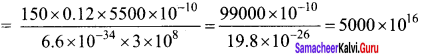n = 5 x 1019

Question 5.
How many photons of frequency 1014 Hz will make up 19.86 J of energy?
Total energy emitted per second = Power x time
19.86 J = Power x is
∴ Power = 19.86 W
Number of photons, n = $$\frac { p }{ E }$$ = $$\frac { p }{ hυ }$$
= $$\frac { 19.86 }{ 6.6\times { 10 }^{ -34 }\times { 10 }^{ 14 } }$$ = 3.009 x 1020
n = 3 x 1020
np = 3 x 1020

Question 6.
What should be the velocity of the electron so that its momentum equals that of 4000 Å wavelength photon.
de-Broglie wavelength of electron
λ = $$\frac { h }{ p }$$
v = $$\frac { h }{ mλ }$$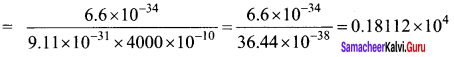v = 1811 ms-1

Question 7.
When a light of frequency 9 x 1014 Hz is incident on a metal surface, photoelectrons are emitted with a maximum speed of 8 x 105ms-1. Determine the threshold frequency of the surface.
According to Einstein’s photoelectric equation
$$\frac { 1 }{ 2 }$$ $${ mv }_{ max }^{ 2 }$$ = h (υ-υ0)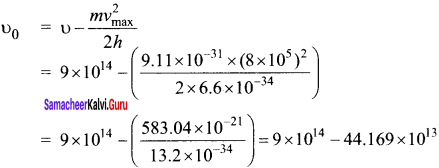= 9 x 014-4.4 x 1014
υ0 = 4.6 x 1014 Hz

Question 8.
When a 6000 Å light falls on the cathode of a photo cell and produced photoemission. If a stopping potential of 0.8 V is required to stop emission of electron, then determine the:

1. frequency of the light
2. energy of the incident photon
3. work function of the cathode material
4. threshold frequency
5. net energy of the electron after it leaves the surface.

Wavelength, λ = 6000 Å= 6000 x 10-10 m
stopping potential, V0 = 0.8 V
1. Frequency of the light, υ = $$\frac { c }{ λ }$$
= $$\frac { 3\times { 10 }^{ 8 } }{ 600\times { 10 }^{ -10 } }$$ = 5 x 104 x 10-18
υ = 5 x 1014 Hz

2. Energy of the incident photon,
E = hυ = 6.6 x 10-34 x 5 x 1014
= 33 x 10-20 J
= $$\frac { 33\times { 10 }^{ -20 } }{ 1.6\times { 10 }^{ -19 } }$$ = 20.625 x 10-1
E = 2.06 eV

3. Work function of the cathode material.
W0 = hυ – eV0
= $$\left(\frac{6.6 \times 10^{-34} \times 5 \times 10^{14}}{1.6 \times 10^{-19}}\right)$$ – $$\left(\frac{1.6 \times 10^{-19} \times 0.8}{1.6 \times 10^{-19}}\right)$$ = 2.06-0.8
W0 = 1.26 eV

4. Threshold frequency, W0 = hυ0
υ0 = $$\frac{W_{0}}{h}$$ = $$\frac{1.26 \times 1.6 \times 10^{-19}}{6.6 \times 10^{-34}}$$ = 0.3055 x 1015
υ0 = 3.05 x 1014 Hz

5. Net energy of the electron after it leaves the surface
E = (υ – υ0)
= 6.6 x 10-34 (5 x1014 – 3.06 x 1014
= 6.6 x 10-34 x 1.94 x 1014
E = 12.804 x 10-20 J
= $$\frac{1.2804 \times 10^{-19}}{1.6 \times 10^{-19}}$$
E = 0.8 e V

Question 9.
A 3310 Å photon liberates an electron from a material with energy 3 x 10-19 J while another 5000 Å photon ejects an electron with energy 0.972×10-19 J from the same material. Determine the value of Planck’s constant and the threshold wavelength of the material.
They energy of ejected electron is given by E = $$\frac { hc }{ λ }$$ – $$\frac { hc }{ { \lambda }_{ 0 } }$$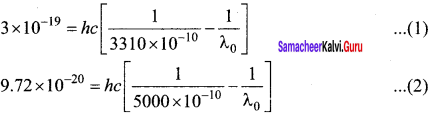Subtracting (2) from (1), we get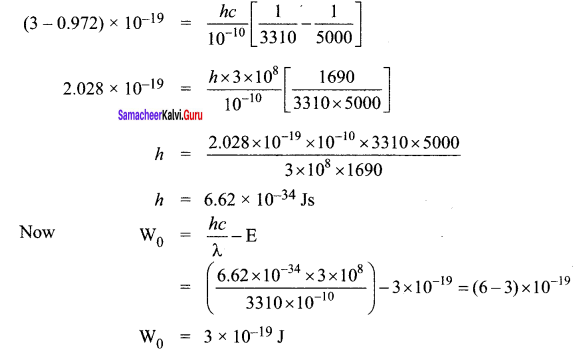Threshold Wavelength,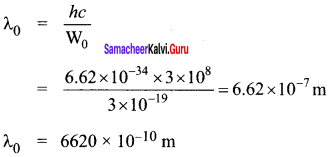Question 10.
At the given point of time, the earth receives energy from Sun at 4 cal cm-2 min-1. Determine the number of photons received on the surface of the Earth per cm2 per minute. (Given : Mean wavelength of Sun light = 5500 Å)
E= 4 calorie
= 4 x 4.184 J
λ = 5500 Å
Number of photons received on the surface of the earth, from E = nhυ
n = $$\frac { E λ}{ hc }$$
= $$\frac{4 \times 4.184 \times 5500 \times 10^{-10}}{6.6 \times 10^{-34} \times 3 \times 10^{8}}$$ = $$\frac{9.2048 \times 10^{-10}}{19.8 \times 10^{-26}}$$ = 4648 x 1016
= 4.648 x 1019
n = 4.65 x 1019

Question 11.
UV light of wavelength 1800 Å is incident on a lithium surface whose threshold wavelength 4965 Å. Determine the maximum energy of the electron emitted.
λ = 1800 x 10-10 m
λ0 = 4965 x 10-10m
h = 6.6 x 10-34 Js
c = 3 x 108 ms-1
Maximum kinetic energy of electron,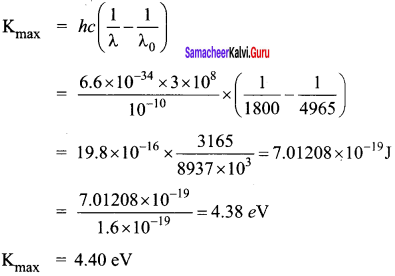Question 12.
Calculate the de Broglie wavelength of a proton whose kinetic energy is equal to 81.9 x 10-15 J. (Given: mass of proton is 1836 times that of electron).
mp = 1.67 x 10-27 kg
K.E = 81.9 x 10-15 J
de-Broglie wavelength of proton, λ = $$\frac { h }{ \sqrt { 2mK } }$$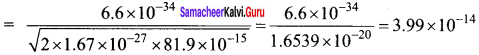λ = 4 x 10-14 m

Question 13.
A deuteron and an alpha particle are accelerated with the same potential. Which one of the two has (i) greater value of de Broglie wavelength associated with it and (ii) less kinetic energy? Explain.
(i) Using de-Broglie wavelength formula, the dueteron and alpha particle are accelerated with same potential. So, both their velocities are same.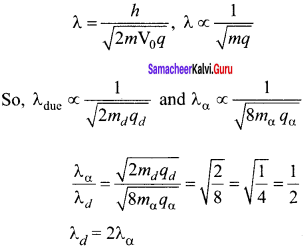(ii) For same potential of acceleration, KE is directly proportional to the ‘q’
Charge of duetron is +e
Charge of alpha is +2e
So, Kd = $$\frac {{ K }_{α}}{ 2 }$$
Charge of alpha particle is more than the duetron.

Question 14.
An electron is accelerated through a potential difference of 81V. What is the de Broglie wavelength associated with it? To which part of electromagnetic spectrum does this wavelength correspond?
de-Broglie wavelength of an electron beam accelerated through a potential difference of V volts is
λ = $$\frac { h }{ \sqrt { 2meV } }$$ = $$\frac { 1.23 }{ \sqrt { V } }$$ nm
V = 81 V, so λ = $$\frac { 1.23 }{ \sqrt { 81 } }$$ x 10-9 m
λ = 1.36 Å
X-ray is the part of electromagnetic spectrum does this wavelength corresponds. X-ray has the wavelengths ranging from about 108 to 10-12 m.

Question 15.
The ratio between the de Broglie wavelengths associated with protons, accelerated through a potential of 512 V and that of alpha particles accelerated through a potential of X volts is found to be one. Find the value of X.
de-Broglie wavelength of accelerated charge particle
λ = $$\frac { h }{ \sqrt { 2mqV } }$$
λ ∝ $$\frac { h }{ \sqrt { mqV } }$$
Ratio of wavelength of proton and a-particle.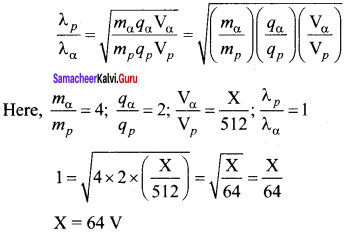### Samacheer Kalvi 12th Physics Dual Nature of Radiation and Matter Additional Questions

Samacheer Kalvi 12th Physics Dual Nature of Radiation and Matter Multiple Choice Questions

Question 1.
Two photons, each of energy 2.5 eV are simultaneously incident on sodium metal surface. If the work function of sodium is 2.75 eV, then from its surface will be emitted.
a) one electron
b) two-electron
c) more than two electrons
d) not a single electron
d) not a single electron

Question 2.
If an electron and proton are propagating in the form of waves having the same λ, it implies that they have the same-
(a) energy
(b) momentum
(c) velocity
(d) angular momentum
(b) momentum
Hint: Momentum, p = $$\frac { h }{ λ }$$
As both electron and proton have same λ, so they have the same momentum

Question 3.
An electron of mass m and charge e is accelerated from rest through a potential difference V in vacuum. Its final velocity will be-
(a) $$\sqrt { \frac { 2eV }{ m } }$$
(b) $$\sqrt { \frac { eV }{ m } }$$
(c) $$\frac { ev }{ 2m }$$
(d) $$\frac { ev }{ m }$$
(a) $$\sqrt { \frac { 2eV }{ m } }$$
Hint:
K.E. gained by an electron when accelerated through a potential difference V,
$$\frac { 1 }{ 2 }$$ mv2 = eV or v = $$\sqrt { \frac { 2eV }{ m } }$$

Question 4.
Scanning electron microscopes are example for _______.
a) Thermionic emission
b) Field emission
c) Photoelectric emission
d) Secondary emission
b) field emission

Question 5.
Sodium and copper have work functions 2.3 eV and 4.5 eV respectively. Then, the ratio of their threshold wavelength is nearest to-
(a) 1 : 2
(b) 4 : 1
(c) 2 : 1
(d) 1 : 4
(c) 2 : 1
Hint:
$$\frac{\lambda_{0}(\mathrm{Na})}{\lambda_{0}(\mathrm{Cu})}$$ = $$\frac{\mathrm{W}_{0}(\mathrm{Cu})}{\mathrm{W}_{0}(\mathrm{Na})}$$

Question 6.
The surface of a metal is illuminated with a light of 400 nm. The kinetic energy of the ejected photoelectrons was found to be 1.68 eV. The work function of the metal is (hc = 1240 eV nm)
(a) 3.09 eV
(b) 1.41 eV
(c) 1.51 eV
(d) 1.68 eV
(b) 1.41 eV
Hint:
Kmax = $$\frac { hc }{ λ }$$ W0 or W0 = $$\frac { hc }{ λ }$$ – Kmax
= $$\frac { 1240 }{ 400 }$$-1.68 = 3.10-1.68 = 1.42ev

Question 7.
The Threshold wavelength for a metal surface whose photoelectric work function is 2.063 eV.
a) 4125 Å
b) 3750 Å
c) 6000 Å
d) 2062 Å
c) 6000 Å

Question 8.
A light having a wavelength of 300 nm falls on a metal surface work function of the metal is 2.54 eV. What is stopping potential?
(a) 1.4 V
(b) 2.59 V
(c) 1.60 V
(d) 1.29 V
(a) 1.4 V
Hint:
eV0 = hu – W0 = 2eV – 0.6 eV = 1.4 eV
∴ V0= $$\frac { 1.4eV }{ e }$$ = 1.4eV

Question 9.
If the kinetic energy of a free electron doubles, its de-Broglie wavelength changes by the factor
(a) $$\frac { 1 }{ 2 }$$
(b) 2
(c) $$\frac { 1 }{ √2 }$$
(d) √2
(c) $$\frac { 1 }{ √2 }$$
Hint:
λ = $$\frac{h}{\sqrt{2 m \mathrm{K}}}$$
When kinetic energy is doubled, λ’ = $$\frac{h}{\sqrt{2 m \times 2 K}}$$ = $$\frac { 1 }{ √2 }$$λ

Question 10.
If the kinetic energy of a particle is increased by 16 times, the percentage change in the de-Broglie wavelength of the particle is
(a) 25%
(b) 75%
(c) 60%
(d) 50%
(b) 75%
Hint:
λ = $$\frac{h}{\sqrt{2 m \mathrm{K}}}$$ ; $$\frac{h}{\sqrt{2 m \times 16 K}}$$ = $$\frac { λ }{ 4 }$$
% change in de-Broglie wavelength, $$\frac { λ-λ’ }{ λ }$$ = [1-$$\frac { λ }{ λ’ }$$] x 100 [1-$$\frac { 1 }{ 4 }$$] x 100 = 75%

Question 11.
When an electron jumps from the M shell to the k shell it gives _______.
a) Kα line
b) Kβ line
c) Lα line
d) Lβ line
b) Kβ line

Question 12.
The kinetic energy of an electron, which is accelerated in the potential difference of 100 volts, is
(a) 416.6 cal
(b) 6.636 cal
(c) 1.602 x 10-17 J
(d) 1.6 x 104 J
(c) 1.602 x 10-17 J
Hint:
K = eV = 1.602 x 10(c) 1.602 x 10-19 x 100 J
= 1.602 x 10(c) 1.602 x 10-17 J

Question 13.
Kinetic energy of emitted electron depends upon
(a) frequency
(b) intensity
(c) nature of atmosphere surrounding the electron
(d) none of these
(a) frequency
Hint:
The kinetic energy of emitted electrons depends on the frequency of incident radiation.

Question 14.
The work function of photo metal is 6.626 eV. What is the threshold wavelength?
(a) 3921 Å
(b) 1875 Å
(c) 1867 Å
(d) 4433 Å
(b) 1875 Å
Hint:
λ0 = $$\frac { hc }{{ W }_{ 0 }}$$ = $$\frac{6.63 \times 10^{-34} \times 3 \times 10^{8} \times 10^{10}}{6.626 \times 1.6 \times 10^{-19}}$$ Å = 1875 Å

Question 14.
The work function of caesium is 1.8 ev then its threshold frequency is ________.
a) 4.36 × 1014 HZ
b) 1.42 × 1014 HZ
c) 8 × 1014 HZ
d) 1.1 × 1015 HZ
a) 4.36 × 1014 HZ
Solution:
W = hν0
= $$\frac{1.8 \times 1.6 \times 10^{-19}}{6.6 \times 10^{-34}}$$
ν0 = 4.36 × 1014 Hz

Question 16.
The speed of an electron having a wavelength of 10-10 m is
(a) 7.25 x 106 ms-1
(b) 6.26 x 106 ms-1
(c) 5.25 x 106 ms-1
(d) 4.24 x 106 ms-1
(a) 7.25 x 106 ms-1
Hint:
As λ = $$\frac { h }{ mv }$$
∴ v = $$\frac { h }{ mλ }$$ = $$\frac{6.6 \times 10^{-34}}{9.1 \times 10^{-31} \times 10^{-10}}$$ = 7.25 x 106 ms-1

Question 17.
If an electron and a photon propagate in the form of waves having the same wavelength, it implies that they have the same
(a) energy
(b) momentum
(c) angular momentum
(d) velocity
(b) momentum
Hint:
As both electron and photon have same de-Broglie wavelength (λ = h /p), so they have the same momentum P.

Question 18.
The frequency of photos having energy 26.52 eV is
a) 6.4 × 1015 Hz
b) 6.4 × 10-24 Hz
c) 4 × 1019 Hz
d) 4.6 × 1024 Hz
a) 6.4 × 1015 Hz
Solution:
E = hv
ν = $$\frac{E}{h}$$
= $$\frac{26.52 \times 1.6 \times 10^{-19}}{6.6 \times 10^{-34}}$$
= 6.42 × 1015 Hz

Question 19.
Photon of frequency u has a momentum associated with it. If c is the velocity of radiation, then the momentum is
(a) $$\frac { hυ }{ c }$$
(b) $$\frac { υ }{ c }$$
(c) hυc
(d) $$\frac { h }{ { c }^{ 2 } }$$
(a) $$\frac { hυ }{ c }$$
Hint:
P = $$\frac { E }{ { c }^{ 2 } }$$ = $$\frac { hυ }{ c }$$

Question 20.
The frequency of photon having the energy of 413 eV
a) 1018 HZ
b) 1017 HZ
c) 1016 HZ
d) 1015 HZ
b) 1017 HZ
Solution:
E = hν
ν = $$\frac{E}{h}$$
ν = $$\frac{413 \times 1.6 \times 10^{-19}}{6.6 \times 10^{-34}}$$
ν = 1017 HZ

Question 21.
Cathode rays consist of
(a) photons
(b) electrons
(c) protons
(d) α-particles
(b) electrons

Question 22.
The momentum of photon whose frequency is f is
(a) $$\frac { hf }{ c }$$
(b) $$\frac { hc }{ f }$$
(c) $$\frac { h }{ f }$$
(d) $$\frac { c }{ hf }$$
(a) $$\frac { hf }{ c }$$
Hint:
p = mc = $$\frac { { mc }^{ 2 } }{ { c } }$$ = $$\frac { hf }{ c }$$

Question 23.
Momentum of electron having kinetic energy 2 eV is
a) 7.63 × 10-25 kg ms-1
b) 7.63 × 10-24 kg ms-1
c) 6.63 × 10-25 kg ms-1
d) 6.63 × 10-24 kg ms-1
a) 7.63 × 10-25 kg ms-1

Question 24.
The ratio of the energy of a photon with λ = 150 nm to that with λ = 300 nm is
(a) 2
(b) $$\frac { 1 }{ 4 }$$
(c) 2
(d) $$\frac { 1 }{ 2 }$$
(a) 2
Hint:
$$\frac {{ E }_{ 1 }}{ { E }_{ 2 } }$$ = $$\frac {{ λ }_{ 2 }}{ { λ }_{ 1 } }$$ = $$\frac { 300 }{ 150 }$$ = 2

Question 25.
Photons of 5.5 eV energy fall on the surface of the metal emitting photoelectrons of maximum kinetic energy 4.0 eV. The stopping voltage required for these electrons is
(a) 5.5 V
(b) 1.5 V
(c) 9.5 V
(d) 4.0 V
(d) 4.0 V
Hint:
Stopping potential = $$\frac { { K }_{ max } }{ e }$$ = $$\frac { 4.0ev }{ e }$$ = 4.0V

Question 26.
The wavelength of photon is proportional to (where υ = frequency)
(a) υ
(b) √υ
(c) $$\frac { 1 }{ √υ }$$
(d) $$\frac { 1 }{ υ }$$
(d) $$\frac { 1 }{ υ }$$
Hint:
λ = $$\frac { c }{ υ }$$ i.e., λ ∝ $$\frac { 1 }{ υ }$$

Question 27.
What is the energy of a photon whose wavelength is 6840 Å?
(a) 1.81 eV
(b) 3.6 eV
(c) – 13.6 eV
(d) 12.1 eV
(a) 1.81 eV
Hint:
E = hυ = $$\frac { hc }{ λ }$$ = $$\frac { 12400ev Å }{ 8840 Å }$$ = 1.81 eV

Question 28.
Momentum of photon of wavelength λ is
(a) $$\frac { hυ }{ c }$$
(b) zero
(c) $$\frac { hλ }{{ c }^{ 2 }}$$
(d) $$\frac { hλ }{c}$$
(a) $$\frac { hυ }{ c }$$
Hint:
p = mc = $$\frac {{ mc }^{ 2 }}{c}$$ = $$\frac { hυ }{ c }$$

Question 29.
The momentum of a photon of energy 1 MeV in kg m/s will be
(a) 5 x 10-22
(b) 0.33 x 106
(c) 7 x 10-24
(d) 10-22
(a) 5 x 10-22
Hint:
P = $$\frac { E }{ c }$$ = $$\frac{1 \mathrm{MeV}}{3 \times 10^{8} \mathrm{ms}^{-1}}$$ = $$\frac{1.6 \times 10^{-13} \mathrm{J}}{3 \times 10^{8} \mathrm{ms}^{-1}}$$ = 5.33 x 10-22 Kg ms-1

Question 30.
If we consider electrons and photons of the same wavelength then will have the same
(a) momentum
(b) angular momentum
(c) energy
(d) velocity
(a) momentum
Hint:
As p = h/λ, so electrons and photons having the same wavelength λ will have the same momentum p.

Question 31.
The photoelectric effect can be explained by
(a) corpuscular theory of light
(b) wave nature of light
(c) Bohr’s theory
(d) quantum theory of light
(d) quantum theory of light

Question 32.
Which of the following waves can produce a photoelectric effect?
(a) ultrasound
(b) infrared
(d) X-rays
(d) X-rays
Hint:
Electromagnetic radiation, being of high frequency such as X-rays can produce a photoelectric effect.

Question 33.
Which light when falls on metal will emit photoelectrons?
(d) microwaves
Hint:
Ultraviolet radiation, being of high frequency, can emit photoelectrons from metals.

Question 34.
In the photoelectric effect, the KE of electrons emitted from the metal surface depends upon
(a) intensity of light
(b) frequency of incident light
(c) the velocity of the incident light
(d) both intensity and velocity of light
(b) frequency of incident light
Hint:
The kinetic energy of photoelectrons depends upon the frequency of incident light.

Question 35.
In the photoelectric effect, electrons are ejected from metals, if the incident light has a certain minimum
(a) wavelength
(b) frequency
(c) amplitude
(d) angle of incidence
(b) frequency
Hint:
For photoelectric emission, the incident light must have a certain minimum frequency, called threshold frequency.

Question 36.
The number of ejected photoelectrons increases with increases
(a) in the intensity of light
(b) in wavelength of light
(c) in the frequency of light
(d) never
(a) in the intensity of light
Hint:
The number of ejected photoelectrons increases with the increase in the intensity of light.

Question 37.
By photoelectric effect, Einstein proved
(a) E = hυ
(b) K.E. = $$\frac { 1 }{ 2 }$$mv2
(c) E = mc2
(d) E = $$\frac {{ -Rhc }^{ 2 }}{{ n }^{ 2 }}$$
(a) E = hυ
Hint:
Einstein explained the photoelectric effect on the basis of Planck’s quantum theory of radiation and hence supported the relation: E = hυ

Question 38.
A photocell employs a photoelectric effect to convert
(a) change in the frequency of light into a change in the electric current
(b) Change in the frequency of light into a change in electric voltage
(c) Change in the intensity of illumination into a change in photoelectric current
(d) Change in the intensity of illumination into a change in the work function of the photocathode
(c) Change in the intensity of illumination into a change in photoelectric current
Hint:
It indicates that threshold frequency is greater than that of ultraviolet light. As X-rays have a greater frequency than UV rays, so they can cause photoelectric effects.

Question 39.
When ultraviolet rays incident on the metal plate their photoelectric effect does not occur, it occurs by the incident of
(a) infrared rays
(b) X-rays
(d) microwave
(b) X-rays
Hint:
It indicates that threshold frequency is greater than that of ultraviolet light. As X-rays have a greater frequency than UV rays, so they can cause a photoelectric effect.

Question 40.
The threshold frequency for the photoelectric effect on sodium corresponds to a wavelength of 5000 Å. Its function is
(a) 4 x 10-19 J
(b) 1J
(c) 2 x 10-19 J
(d) 3 x 10-19 J
(a) 4 x 10-19 J
Hint:
W0 = $$\frac { hc }{{ λ }_{ 0 }}$$ = $$\frac{6.63 \times 10^{-34} \times 3 \times 10^{8}}{5000 \times 10^{-10}}$$ J = 4 x 10-19 J

Question 41.
The photoelectric work function for a metal surface is 4.125 eV. The cut off wavelength for this surface is
(a) 3000 Å
(b) 2062.5 Å
(c) 4125 Å
(d) 6000 Å
(a) 3000 Å
Hint:
λ0 = $$\frac { hc }{{ W }_{ 0 }}$$ = $$\frac{6.63 \times 10^{-34} \times 3 \times 10^{8}}{4.125 \times 1.6 \times 10^{-19}}$$ m = 3 x 10-7 m = 3000 Å

Question 42.
Ultraviolet radiations of 6.2 eV falls on an aluminium surface. Kinetic energy of fastest electrons emitted is (work function = 4.2 eV)
(a) 3.2 x 10-21 J
(b) 3.2 x 10-19 J
(c) 7 x 10-25 J
(d) 9 x 10-32 J
(b) 3.2 x 10-19 J
Hint:
Kmax = hυ- W0 = 6.2 eV – 4.2 eV
= 2.0 eV = 2.0 x 1.6 x 10-19 J =3.2 x 10-19 J

Question 43.
The de-Broglie wavelength of a tennis ball of mass 60g moving with a velocity of 10 ms-1 is approximately (planck’s constant, h = 6.63 x 10-34 Js)
(a) 10-33 m
(b) 10-31 m
(c) 10-16 m
(d) 10-25 m
(a) 10-33 m
Hint:
λ = $$\frac { h }{ mv }$$ = $$\frac{6.63 \times 10^{-34}}{60 \times 10^{-3} \times 10}$$ ≈ 10-33 m

Question 44.
The wavelength of de-Broglie wave is 2 μm, then its momentum (h = 6.63 x 10-34 Js) is
(a) 3.315 x 10-28 kg ms-1
(b) 1.66 x 10-28 kg ms-1
(c) 4.97 x 10-28 kg ms-1
(d) 9.9 x 10-28 kg ms-1
(a) 3.315 x 10-28 kg ms-1
Hint:
p = $$\frac { h }{ λ }$$ = $$\frac{6.03 \times 10^{-34} \mathrm{Js}}{2 \times 10^{-6} \mathrm{m}}$$ = 3.315 x 10-28 kg ms-1

Question 45.
What is de-Broglie wavelength of electron having energy 10 KeV?
(a) 0.12 Å
(b) 1.2 Å
(c) 12.2 Å
(d) none of these
(a) 0.12 Å
Hint:
λ = $$\frac { 12.3 }{ √v }$$ Å = $$\frac { 12.3 }{ \sqrt { 10\times { 10 }^{ 3 } } }$$ = 0.12Å

Question 46.
Which one of the following properties do not support the wave theory of light?
(a) Light obeys laws of reflection and refraction
(b) Light waves get polarised
(c) Light shows the photoelectric effect
(d) Light shows interference
(c) Light shows the photoelectric effect
Hint:
The photoelectric effect cannot be explained on the basis of the wave theory of light.

Question 47.
de-Broglie wavelength λ associated with neutrons is related with absolute temperature T as
(a) λ ∝ T
(b) λ ∝ $$\frac { 1 }{ T }$$
(c) λ ∝ $$\frac { 1 }{ √T }$$
(d) λ ∝ T2
(c) λ ∝ $$\frac { 1 }{ √T }$$
Hint:
λ = $$\frac { h }{ \sqrt { 2mK } }$$ = $$\frac { h }{ \sqrt { 3mKT } }$$ ⇒ λ ∝ $$\frac { 1 }{ √T }$$

Question 48.
As the intensity of incident light increases
(a) kinetic energy of emitted photoelectrons increases
(b) photoelectric current decreases
(c) photoelectric current increases
(d) kinetic energy of emitted photoelectrons decreases
(c) photoelectric current increases
Hint:
As the intensity of incident light increases, photoelectric current increases.

Question 49.
The de Broglie wave corresponding to a particle of mass m and velocity u has a wavelength associated with it
(a) $$\frac { h }{ mυ }$$
(b) hmυ
(c) $$\frac { mh }{ υ }$$
(d) $$\frac { m }{ hυ }$$
(a) $$\frac { h }{ mυ }$$
Hint:
de-Broglie wavelength, λ = $$\frac { h }{ p }$$ = $$\frac { h }{ mυ }$$

Question 50.
If particles are moving with same velocity, then which has maximum de-broglie wavelength?
(a) Proton
(b) α-particle
(c) Nevtron
(d) β-particle
(d) β-particle
Hint:
As λ = h/mv, of the given particles β – particle is the lightest, so it will have a maximum de-Broglie wavelength.

Question 51.
The dual nature of light is exhibited by
(a) diffraction and the photoelectric effect
(b) photoelectric effect
(c) refraction and interference
(d) diffraction and reflection
(a) diffraction and the photoelectric effect
Hint:
Diffraction exhibits wave nature while photoelectric effect exhibits particle nature. Hence these two phenomena exhibit the dual nature of light.

Question 52.
If the momentum of a particle is doubled, then its de-Broglie wavelength will-
(a) remain unchanged
(b) become four-time
(c) become two times
(d) become half
(d) become half
Hint:
As λ = $$\frac { h }{ p }$$ when momentum p is doubled, wavelength will become half the initial value.

Question 53.
Moving with the same velocity, which of the following has the longest de-Broglie wavelength?
(a) β – particle
(b) α – particle
(c) proton
(d) neutron
(a) β – particle
Hint:
λ = $$\frac { h }{ mv }$$ λ ∝ $$\frac { 1 }{ m}$$
As β – particle (an electron) has the smallest mass, so it has the longest de-Broglie wavelength.

Question 54.
What is the de-Broglie wavelength of the a-particle accelerated through a potential difference of V volt? (mass of a-particle = 6.6455 x 10-27 kg)
(a) $$\frac { 0.287 }{ √V }$$ Å
(b) $$\frac { 12.27 }{ √V }$$ Å
(c) $$\frac { 0.101 }{ √V }$$ Å
(d) $$\frac { 0.202 }{ √V }$$ Å
(c) $$\frac { 0.101 }{ √V }$$ Å
Hint:
K = qV = 2eV
λ = $$\frac { h }{ \sqrt { 2mK } }$$ = $$\frac { h }{ \sqrt { 2m\times 2eV\quad } }$$ = $$\frac { h }{ \sqrt { 4meV } }$$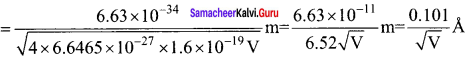Question 55.
A proton and an a – particle are accelerated through the same potential difference. The ratio of de-Broglie wavelength of proton to the de-Broglie wavelength of alpha particle will be
(a) 1 : 2
(b) 2√2 :1
(c) 2 : 1
(d) 1:1
(b) 2√2 :1
Hint: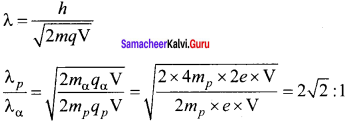Question 56.
Proton and α – particle have the same de-Broglie wavelength. What is same for both of them?
(a) Time period
(b) Energy
(c) Frequency
(d) Momentum
(d) Momentum
Hint:
λ = h/p, when wavelength λ is same, momentump is also same.

Question 57.
The shortest wavelength of X-ray emitted from an X-ray tube depends upon.
(a) the current in the tube
(b) the voltage applied to the tube
(c) the nature of the gas in the tube
(d) the atomic number of the target material
(b) the voltage applied to the tube
Hint:
λmin = $$\frac { hc }{ eV }$$ i.e.,λmin ∝ $$\frac { 1 }{ V }$$

Question 58.
An X-ray tube operates on 30 kV. The minimum wavelength emitted is h = 6.6 x 10-34 Js, c = 3 x 108 m/s, e = 1.6 x 10-19C.
(a) 6.6 Å
(b) 0.133 Å
(c) 1.2 Å
(d) 0.4 Å
(d) 0.4 Å
Hint:
λmin = $$\frac { hc }{ eV }$$ = $$\frac{6.6 \times 10^{-34} \times 3 \times 10^{8}}{1.6 \times 10^{-19} \times 30 \times 10^{3}}$$ m = 0.4 Å

Question 59.
The potential difference between the cathode and the target in a coolidge tube is 120 kV. What can be the minimum wavelength (in Å) of the X-rays emitted by this tube?
(a) 0.4 Å
(b) 0.3 Å
(c) 0.2 Å
(d) 0.1 Å
(d) 0.1 Å
Hint:
λmin = $$\frac { 12375 }{ V }$$ = Å = $$\frac { 12375 }{{ 120×10 }^{3}}$$ Å = 0.1Å

Question 60.
The work function for Al, K and Pt is 4.28 eV, 2.30 eV and 5.65 eV respectively. Their respective threshold frequencies would be
(a) pt > AL > K
(b) Al > pt > K
(c) K > AL > pt
(d) Al > K > pt
(a) pt > AL > K
Hint:
As W0 = hv0 i.e., W0 ∝ V0
V0 (pt) >0 (AL) >V0 (K)

Question 61.
Among the following four spectral regions, the photons has the highest energy in
(a) Infrared
(b) Violet
(c) Red
(d) Blue
(b) Violet
Hint:
E = $$\frac { hc }{ λ }$$ Photon in violet region has least λ and hence highest energy.

Samacheer Kalvi 12th Physics Dual Nature of Radiation and Matter Short Answer Questions

Question 1.
Define electron volt. Express its value in joule.
It is the kinetic energy gained by an electron when it is accelerated through a potential difference of 1 volt.
1 eV = 1.6 x 10-19 J
1 MeV = 1.6 x 10-13J

Question 2.
Define stopping potential.
Stopping potential is that the values of the negative (retarding) potential given to the collecting electrode A which is just sufficient to stop the most energetic photoelectrons emitted and make the photocurrent Zero.

Question 3.
Define the term ‘stopping potential’ in relation to the photoelectric effect.
The minimum negative potential given to the anode of a photo-cell for which the photoelectric current becomes zero is called stopping potential.

Question 4.
Give some important uses of photo-cells.
Applications of photocells:

1. Photocells have many applications, especially as switches and sensors.
2. Automatic lights that turn on when it gets dark use photocells, as well as street lights that switch on and off according to whether it is night or day.
3. Photocells are used for the reproduction of sound in motion pictures and are used as timers to measure the speeds of athletes during a race.

Question 5.
What are characteristic x-ray spectra?
x-ray spectra show more narrow peaks at some well-defined wavelength when the target is hit by fast electrons. The line spectrum showing these peaks are called the characteristic x-ray spectrum.

Question 6.
On what principle is an electron microscope-based?
An electron microscope exploits the wave nature of an accelerated beam of electrons (having a very small wavelength) to provide high magnifying and resolving powers.

Question 7.
What is a photovoltaic cell?
Any sensitive element made of semiconductors is used which generates a voltage which is proportional to the intensity of light or other radiations.

Samacheer Kalvi 12th Physics Dual Nature of Radiation and Matter Long Answer Questions

Question 1.
Describe an experimental arrangement to study the photoelectric effect.
Experimental setup:
1.  The apparatus is employed to study the phenomenon of the photoelectric effect in detail.

2.  S is a source of electromagnetic waves of known and variable frequency v and intensity I. C is the cathode (negative electrode) made up of photosensitive material and is used to emit electrons.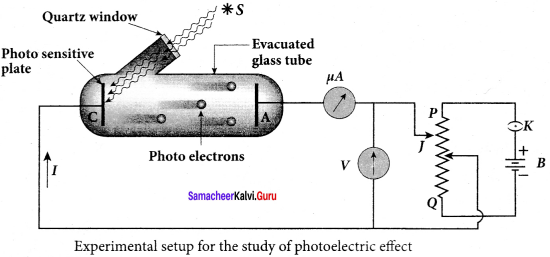3. The anode (positive electrode) A collects the electrons emitted from C. These electrodes are taken in an evacuated glass envelope with a quartz window that permits the passage of ultraviolet and visible light.

4. The necessary potential difference between C and A is provided by high tension battery B which is connected across a potential divider arrangement PQ through a key K. C is connected to the centre terminal while A to the sliding contact J of the potential divider.

5. Plate A can be maintained at a desired positive or negative potential with respect to C. To measure both the positive and negative potential of A with respect to C, the voltmeter is designed to have its zero marking at the centre and is connected between A and C. The current is measured by a microammeter μA in series.

6. If there is no light falling on the cathode C, no photoelectrons are emitted and the microammeter reads zero. When ultraviolet or visible light is allowed to fall on C, the photoelectrons are liberated and are attracted towards the anode.

7. As a result, the photoelectric current is set up in the circuit which is measured using a microammeter.

8.  The variation of photocurrent with respect to-

• the intensity of incident light
• the potential difference between the electrodes
• the nature of the material and
• frequency of incident light can be studied with the help of this apparatus.

Question 2.
Derive the Duane-Hunt formula for continuous X-rays. Can this be explained by the classical electromagnetic theory?
1. The energy of the photon emitted is equal to the loss of kinetic energy of the electron.
2. The photons are emitted with all possible energies.
3. The photon is emitted with the highest frequency ν0.
4. Initial kinetic energy = eV
5. V-accelerating voltage
∴ hν0 = eV
$$\frac{\mathrm{hc}}{\lambda_{0}}$$ = eV
λ0 = $$\frac{\mathrm{hc}}{\mathrm{eV}}$$
λ0 = cut-off wavelength
6. Substituting the known values we get
λ0 = $$\frac{12400}{\mathrm{v}}$$ Å

7. This equation is known as the Duane- Hunt formula.
8. This cannot be explained by the classical electromagnetic theory but explained on the basis of the photon theory of radiation.

Question 3.
Briefly explain the nature of light, (wave-particle duality)
The nature of light: wave-particle duality
We have learned that the wave nature of light explains phenomena such as interference, diffraction, and polarization. Certain phenomena like black body radiation, photoelectric effect can be explained by assigning particle nature to light. Therefore, both theories have enough experimental evidence.

In the past, many scientific theories have been either revised or discarded when they contradicted new experimental results. Here, two different theories are needed to answer the question: what is the nature of light?
It is therefore concluded that light possesses dual nature, that of both particle and wave. It behaves like a wave at some circumstances and it behaves like a particle in some other circumstances.

In other words, light behaves as a wave during its propagation and behaves as a particle during its interaction with matter. Both theories are necessary for a complete description of physical phenomena. Hence, the wave nature and quantum nature complement each other.

Question 4.
Derive de-Broglie wave equation (wavelength) for a material particle.
De Broglie wave length:
The momentum of photon of frequency v is given by
p = $$\frac { hυ }{ c }$$ = $$\frac { h }{ λ }$$ since c = υλ
The wavelength of a photon in terms of its momentum is
λ = $$\frac { h }{ p }$$ …(1)
According to de Broglie, the above equation is completely a general one and this is applicable to material particles as well. Therefore, for a particle of mass m travelling with speed v , the wavelength is given by
λ = $$\frac { h }{ mv }$$ = $$\frac { h }{ p }$$ ….. (2)
This wavelength of the matter waves is known as the de Broglie wavelength. This equation relates the wave character (the wavelength λ) and the particle character (the momentum p) through Planck’s constant.

Question 5.
Explain the production of X-rays.
Production of x-rays:
X-rays are produced in x-ray tube which is essentially a discharge tube. A tungsten filament F is heated to incandescence by a battery. As a result, electrons are emitted from it by thermionic emission.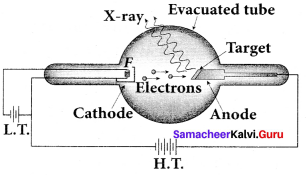The electrons are accelerated to high speeds by the voltage applied between the filament F and the anode. The target materials like tungsten, molybdenum are embedded in the face of the solid copper anode. The face of the target is inclined at an angle with respect to the electron beam so that x-rays can leave the tube through its side.

When high-speed electrons strike the target, they are decelerated suddenly and lose their kinetic energy. As a result, x-ray photons are produced. Since most of the kinetic energy of the bombarding electrons gets converted into heat, targets made of high-meltmg-point metals and a cooling system are usually employed.

Question 6.
Briefly explain the concept of continuous X-ray spectra.
Continuous x-ray spectra:
When a fast-moving electron penetrates and approaches a target nucleus, the interaction between the electron and the nucleus either accelerates or decelerates it which results in a change of path of the electron. The radiation produced from such decelerating electron is called Bremsstrahlung or braking radiation.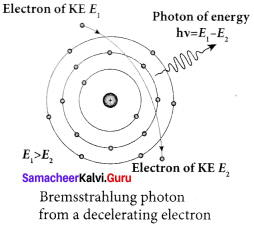The energy of the photon emitted is equal to the loss of kinetic energy of the electron. Since an electron may lose part or all of its energy to the photon, the photons are emitted with all possible energies (or frequencies). The continuous x-ray spectrum is due to such radiations.

When an electron gives up all its energy, then the photon is emitted with highest frequency υ0 (or lowest wavelength λ0 ). The initial kinetic energy of an electron is given by eV where V is the accelerating voltage. Therefore, we have
0 = eV (or) $$\frac { hc }{{ λ }_{0}}$$ = ev
λ0 = $$\frac { hc }{eV}$$
where λ0 is the cut-off wavelength. Substituting the known values in the above equation, we get
λ0 = $$\frac { 122400 }{V}$$ Å
The relation given by the equation is known as the Duane – Hunt formula.
The value of λ0 depends only on the accelerating potential and is the same for all targets. This is in good agreement with the experimental results. Thus, the production of continuous x-ray spectrum and the origin of cut-off wavelength can be explained on the basis of the photon theory of radiation.

Question 7.
Write down the applications of X-rays.
Applications of x-rays:
X-rays are being used in many fields. Let us list a few of them.
1. Medical diagnosis:
X-rays can pass through the flesh more easily than through bones. Thus an x-ray radiograph containing a deep shadow of the bones and a light shadow of the flesh may be obtained. X-ray radiographs are used to detect fractures, foreign bodies, diseased organs, etc.

2. Medical therapy:
Since x-rays can kill diseased tissues, they are employed to cure skin diseases, malignant tumours etc.

3. Industry:
X-rays are used to check for flaws in welded joints, motor tyres, tennis balls, and wood. At the customs post, they are used for the detection of contraband goods.

4. Scientific research:
X-ray diffraction is an important tool to study the structure of crystalline materials – that is, the arrangement of atoms and molecules in crystals.

Samacheer Kalvi 12th Physics Dual Nature of Radiation and Matter Additional Numerical Problems

Question 1.
If a light of wavelength 4950 Å is viewed as a continuous flow of photons, what is the energy of each photon in eV? (Given h = 6.6 x 10-34 Js, c = 3 x 108 ms-1)
Solution:
Here λ = 4950 Å = 4950 x 10-10 m
Energy of each photon,
E = $$\frac { hc }{λ}$$ = $$\frac{6.6 \times 10^{-34} \times 3 \times 10^{8}}{4950 \times 10^{-10}}$$ = 4 x 10-19 J
= $$\frac{4 \times 10^{-19}}{1.6 \times 10^{-19}}$$eV
E = 2.5 eV

Question 2.
Calculate the threshold wavelength of certain metal of work function 1.8 eV.
Work function: W = hν0
ν0 = $$\frac{W}{h}$$
threshold wavelength λ0 = $$\frac{c}{v_{o}}=\frac{c h}{w}$$
= $$\frac{19.872}{2.88}$$ × 10-26 × 1019
= 6.9 × 10-7
λ = 6900 Å

Question 3.
Light of wavelength 5000 Å falls on a metal surface of work function 1.9 eV. Find:
(i) the energy of photons in eV
(ii) the K.E of photoelectrons and
(iii) the stopping potential.
Solution:
Here λ = 5000 Å = 5 x 10-7 m
W0 = 1.9 ev
(i) Energy of a photon,
E = $$\frac { hc }{λ}$$ = $$\frac{6.6 \times 10^{-34} \times 3 \times 10^{8}}{5 \times 10^{-7}}$$ J = $$\frac{6.6 \times 10^{-34} \times 3 \times 10^{8}}{5 \times 10^{-7} \times 1.6 \times 10^{-19}} e V$$ eV
E = 2.475 eV
(ii) K.E of a photoelectron,
K.E = hυ – W0 = 2.475 – 1.9 = 0.575 eV
(iii) Let V0 be the stopping potential. Then
eV0 = $$\frac { 1 }{ 2 }$$ mv2 = K.E of a photoelectron
V0 = $$\frac { 0.575 }{ e }$$ eV
V0 = 0.575 V

Question 4.
If photoelectrons are to be emitted from a potassium surface with a speed 6 x 106 ms-1, what frequency of radiation must be used? (Threshold frequency for potassium is 4.22 x 1014 Hz, h = 6.6 x 10-34 Js, me = 9.1 x 10-31 kg)
Solution:
Here, v = 6 x 106 ms-1
V0 = 4.22 x 1014 Hz
From Einstein’s photoelectric equation,
k.E = $$\frac { 1 }{ 2 }$$ mv2 = h (υ – υ0)
υ = $$\frac { 1 }{ 2 }$$ $$\frac {{ mv }^{2}}{ h }$$ + υ0
= $$\frac { 1 }{ 2 }$$ x $$\frac{9.1 \times 10^{-31}+\left(6 \times 10^{6}\right)^{2}}{6.6 \times 10^{-34}}$$ + 4. 22 x 10-14
= (2.48 x 1014) + (4. 22 x 1014)
υ = 6.7 x1014 Hz

Question 5.
The photoelectric cut-off voltage in a certain experiment 1.5 V. What is the maximum kinetic energy of photoelectrons emitted?
Solution:
Here V0 = 1.5 V
Kmax = eV0 = 1.5 eV
= 1.5 x 1.6 x 10-19 J
Kmax = 24 x 10-19 J

Question 6.
When the light of wavelength 2200 Å falls non Cu, photoelectrons are emitted from it. Find
(i) the threshold wavelength and
(ii) the stopping potential (work function for Cu is Φ0 = 4.65 eV)
(i) The threshhold wavelength is given by
λ0 = $$\frac{h c}{\phi_{0}}$$
= $$\frac{6.626 \times 10^{-34} \times 3 \times 10^{8}}{4.65 \times 1.6 \times 10^{-19}}$$
= 2672 Å

(ii) Energy of the photon of wavelength 2200 Å is
E = $$\frac{h c}{\lambda}$$
= $$\frac{6.626 \times 10^{-34} \times 3 \times 10^{8}}{2200 \times 10^{-10}}$$
= 9.035 × 10-19 J = 5.65 eV
We know that kinetic energy of fastest photo electron is
Kmax = hν – Φ0 = 5.65 – 4.65 = 1 eV
From above equation
Kmax = eV0
V0 = $$\frac{K_{\max }}{e}$$
= $$\frac{1 \times 1.6 \times 10^{-19}}{1.6 \times 10^{-19}}$$
Therefore, stopping potential = 1 V

Question 7.
An electron and a photon each have a wavelength of 1 nm. Find, (a) their momenta (b) the energy of the photon, and (c) kinetic energy of the electron.
Solution:
(a) Both electron and photon have same wavelength. so, they have same momentum also,
P = $$\frac { h }{ λ }$$ = $$\frac{6.6 \times 10^{-34}}{1 \times 10^{-9}}$$ = 6.6 x 10-25 kg ms-1
(b) Energy of a photon,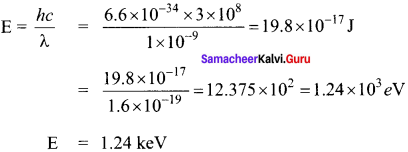(c) Kinetic energy of electron,
K = $$\frac {{ p }^{2}}{ 2m }$$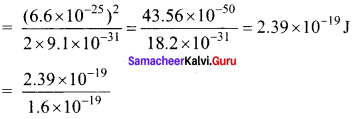K = 1.49 eV

Question 8.
Find the ratio of de-Broglie wavelengths associated with two electron beams accelerated through 25 V and 36 V respectively.
Solution:
de-Broglie wavelength associate with potential difference λ ∝ $$\frac { 1 }{ √V }$$
$$\frac {{ λ }_{1}}{ { λ }_{2} }$$ = $$\sqrt { \frac { { V }_{ 2 } }{ { V }_{ 1 } } }$$ = $$\sqrt { \frac { 36 }{ 25 } }$$ = $$\frac { 6 }{ 5 }$$ ⇒ λ1 : λ2 = 6 : 5

Question 9.
A proton and an alpha particle, both initially at rest, are accelerated so as to have the same kinetic energy. What is the ratio of their de-Broglie wavelength?
Solution:
de-Broglie wavelength,
λ = $$\frac { h }{ p }$$ = $$\frac { h }{ \sqrt { 2mK } }$$
i.e.
λ ∝ $$\frac { 1 }{ √m}$$ [mα = 4mp]
$$\frac {{ λ }_{p}}{ { λ }_{α} }$$ = $$\sqrt { \frac { { m }_{ α } }{ { m }_{ p } } }$$ = $$\sqrt { \frac { { 4m }_{ p } }{ { m }_{ p } } }$$ = $$\sqrt { \frac { 4 }{ 1 } }$$ = $$\frac { 2 }{ 1 }$$
λp : λα = 2: 1

Question 10.
Light of two different frequencies whose photons have energies 1 eV and 2.5 eV respectively illuminate a metallic surface whose work function is 0.5 eV successively. Find the ratio of maximum speeds of emitted electrons.
Solution: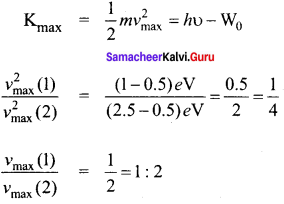Hope the data shared has shed some light on you regarding the Samacheer Kalvi Class 12th Physics Solutions Chapter 7 Dual Nature of Radiation and Matter Questions and Answers. Do leave us your queries via the comment section and we will guide you at the earliest with help seeked. Stay connected with our website and avail the latest information in a matter of seconds.